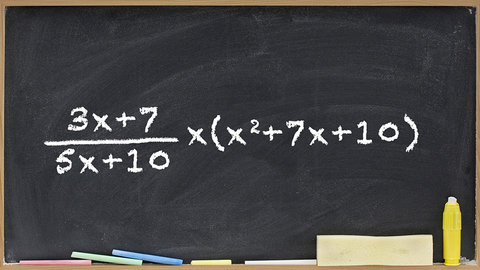# Rational Expressions, Part 2 Episode 29 of Algebra I

##### The Great Courses## Related videos

Rational Expressions, Part 1
Episode 28 of Algebra I
When one polynomial is divided by another, the result is called a rational function because it is the ratio of two polynomials. These functions play an important role in algebra. Learn how to add and subtract rational functions by first finding their common divisor.
Graphing Rational Functions, Part 1
Episode 30 of Algebra I
Examine the distinctive graphs formed by rational functions, which may form vertical or horizontal curves that aren't even connected on a graph. Learn to identify the intercepts and the vertical and horizontal asymptotes of these fascinating curves.
Graphing Rational Functions, Part 2
Episode 31 of Algebra I
Sketch the graphs of several rational functions by first calculating the vertical and horizontal asymptotes, the x and y intercepts, and then plotting several points in the function. In the final exercise, you must simplify the expression in order to extract the needed information.
Variables and Algebraic Expressions
Episode 4 of Algebra I
Advance to the next level of problem solving by using variables as the building blocks to create algebraic expressions, which are combinations of mathematical symbols that might include numbers, variables, and operation symbols. Also learn some tricks for translating the language of problems (phrases in English) into the language of…
Operations and Expressions
Episode 5 of Algebra I
Discover that by following basic rules on how to treat coefficients and exponents, you can reduce very complicated algebraic expressions to much simpler ones. You start by using the commutative property of multiplication to rearrange the terms of an expression, making combining them relatively easy.
Episode 32 of Algebra I
Anytime you see a root symbol - for example, the symbol for a square root - then you're dealing with what mathematicians call a radical. Learn how to simplify radical expressions and perform operations on them, such as multiplication, division, addition, and subtraction, as well as combinations of these operations.
Solving Linear Equations, Part 1
Episode 7 of Algebra I
In this lesson, work through simple one- and two-step linear equations, learning how to isolate the variable by different operations. Professor Sellers also presents a word problem involving a two-step equation and gives tips for how to solve it.
Solving Linear Equations, Part 2
Episode 8 of Algebra I
Investigating more complicated examples of linear equations, learn that linear equations fall into three categories. First, the equation might have exactly one solution. Second, it might have no solutions at all. Third, it might be an identity, which means every number is a solution.
Graphing Linear Equations, Part 1
Episode 10 of Algebra I
Use what you've learned about slope to graph linear equations in the slope-intercept form, y = mx + b, where m is the slope, and b is the y intercept. Experiment with examples in which you calculate the equation from a graph and from a table of pairs of points.
Graphing Linear Equations, Part 2
Episode 11 of Algebra I
A more versatile approach to writing the equation of a line is the point-slope form, in which only two points are required, and neither needs to intercept the y axis. Work through several examples and become comfortable determining the equation using the line and the line using the equation.
Systems of Linear Equations, Part 1
Episode 15 of Algebra I
When two lines intersect, they form a system of linear equations. Discover two methods for finding a solution to such a system: by graphing and by substitution. Then try out a real-world example, involving a farmer who wants to plant different crops in different proportions.
Systems of Linear Equations, Part 2
Episode 16 of Algebra I
Expand your tools for solving systems of linear equations by exploring the method of solving by elimination. This technique allows you to eliminate one variable by performing addition, subtraction, or multiplication on both sides of an equation, allowing a straightforward solution for the remaining variable.